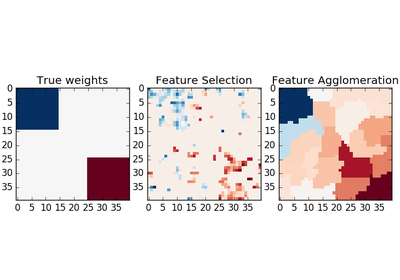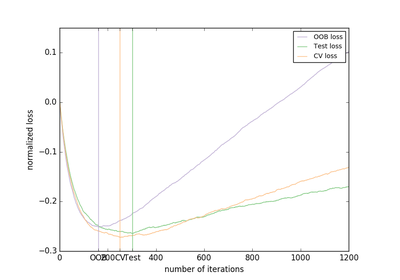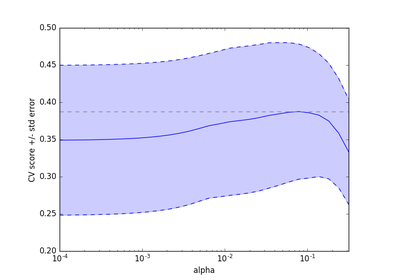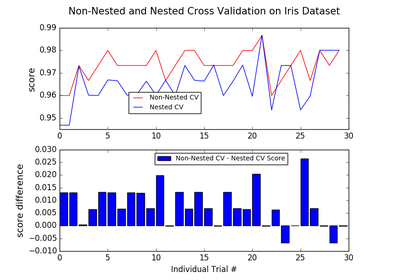# `sklearn.model_selection`.KFold¶

class `sklearn.model_selection.``KFold`(n_splits=3, shuffle=False, random_state=None)[source]

K-Folds cross-validator

Provides train/test indices to split data in train/test sets. Split dataset into k consecutive folds (without shuffling by default).

Each fold is then used once as a validation while the k - 1 remaining folds form the training set.

Read more in the User Guide.

Parameters: n_splits : int, default=3 Number of folds. Must be at least 2. shuffle : boolean, optional Whether to shuffle the data before splitting into batches. random_state : None, int or RandomState When shuffle=True, pseudo-random number generator state used for shuffling. If None, use default numpy RNG for shuffling.

`StratifiedKFold`
Takes group information into account to avoid building folds with imbalanced class distributions (for binary or multiclass classification tasks).
`GroupKFold`
K-fold iterator variant with non-overlapping groups.

Notes

The first `n_samples % n_splits` folds have size `n_samples // n_splits + 1`, other folds have size `n_samples // n_splits`, where `n_samples` is the number of samples.

Examples

```>>> from sklearn.model_selection import KFold
>>> X = np.array([[1, 2], [3, 4], [1, 2], [3, 4]])
>>> y = np.array([1, 2, 3, 4])
>>> kf = KFold(n_splits=2)
>>> kf.get_n_splits(X)
2
>>> print(kf)
KFold(n_splits=2, random_state=None, shuffle=False)
>>> for train_index, test_index in kf.split(X):
...    print("TRAIN:", train_index, "TEST:", test_index)
...    X_train, X_test = X[train_index], X[test_index]
...    y_train, y_test = y[train_index], y[test_index]
TRAIN: [2 3] TEST: [0 1]
TRAIN: [0 1] TEST: [2 3]
```

Methods

 `get_n_splits`([X, y, groups]) Returns the number of splitting iterations in the cross-validator `split`(X[, y, groups]) Generate indices to split data into training and test set.
`__init__`(n_splits=3, shuffle=False, random_state=None)[source]
`get_n_splits`(X=None, y=None, groups=None)[source]

Returns the number of splitting iterations in the cross-validator

Parameters: X : object Always ignored, exists for compatibility. y : object Always ignored, exists for compatibility. groups : object Always ignored, exists for compatibility. n_splits : int Returns the number of splitting iterations in the cross-validator.
`split`(X, y=None, groups=None)[source]

Generate indices to split data into training and test set.

Parameters: X : array-like, shape (n_samples, n_features) Training data, where n_samples is the number of samples and n_features is the number of features. y : array-like, shape (n_samples,) The target variable for supervised learning problems. groups : array-like, with shape (n_samples,), optional Group labels for the samples used while splitting the dataset into train/test set. train : ndarray The training set indices for that split. test : ndarray The testing set indices for that split.

## Examples using `sklearn.model_selection.KFold`¶Feature agglomeration vs. univariate selection